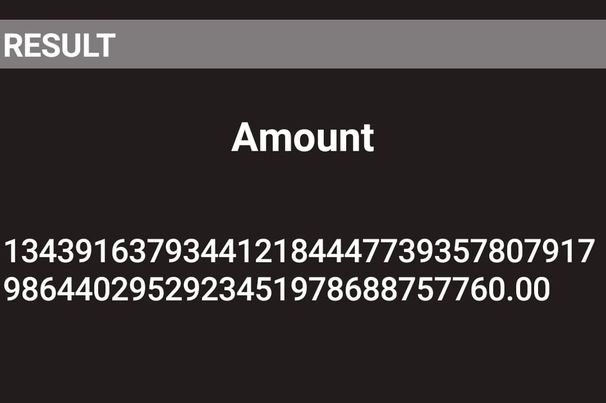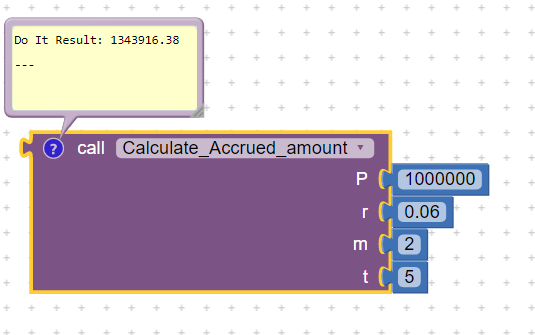# How do I fix the decimal places and how do I remove the zeroes after it?

We are making a Compound Interest Calculator. The decimal point in the result was not in a right place, there were 2 zeroes at the end that make the answer wrong, and instead of getting 1343916.38, the result was 134391637.00 (as shown in the picture). I am just a beginner and I am still exploring. How can I solve this and get the right result? I'd really appreciate it if you will help me now because this is our project for our school.

This is the formula of the Compound Interest

This is the equation: (The answer is supposed to be like this)

but this is the result that I got from my phone (android)

and this is the code (I am still learning how to code)

Maybe this might help

1 Like

Try like this (which matches the Bank of Manila):

1 Like

Thanks but this is the result

and this is the result on my phone (android)1 Like

These formulas are easier to check if you switch their sockets to external mode.

1 Like

Try creating a procedureProcedure is draggable

2 Likes

This topic was automatically closed 7 days after the last reply. New replies are no longer allowed.